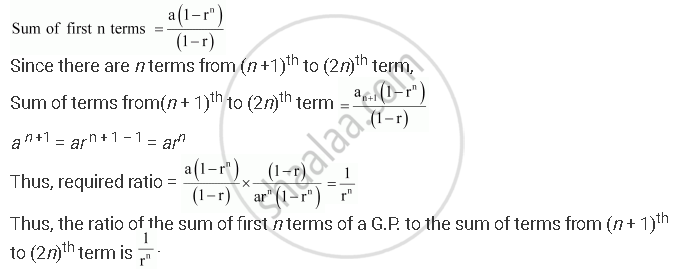CBSE (Arts) Class 11CBSE
Share
Notifications

View all notifications
Books Shortlist
Your shortlist is empty

# Show that the Ratio of the Sum of First N Terms of a Geometric Progression. to the Sum of Terms from (N + 1)^(Th) " to "(2n)^(Th) " Term is " 1/R^N - CBSE (Arts) Class 11 - Mathematics

Login
Create free account

Forgot password?
ConceptGeometric Progression (G. P.)

#### Question

Show that the ratio of the sum of first n terms of a G.P. to the sum of terms from (n + 1)^(th) " to "(2n)^(th) " term is " 1/r^n.

#### Solution

Let a be the first term and be the common ratio of the G.P.Is there an error in this question or solution?

#### APPEARS IN

NCERT Solution for Mathematics Textbook for Class 11 (2018 to Current)
Chapter 9: Sequences and Series
Q: 24 | Page no. 193
Solution Show that the Ratio of the Sum of First N Terms of a Geometric Progression. to the Sum of Terms from (N + 1)^(Th) " to "(2n)^(Th) " Term is " 1/R^N Concept: Geometric Progression (G. P.).
S